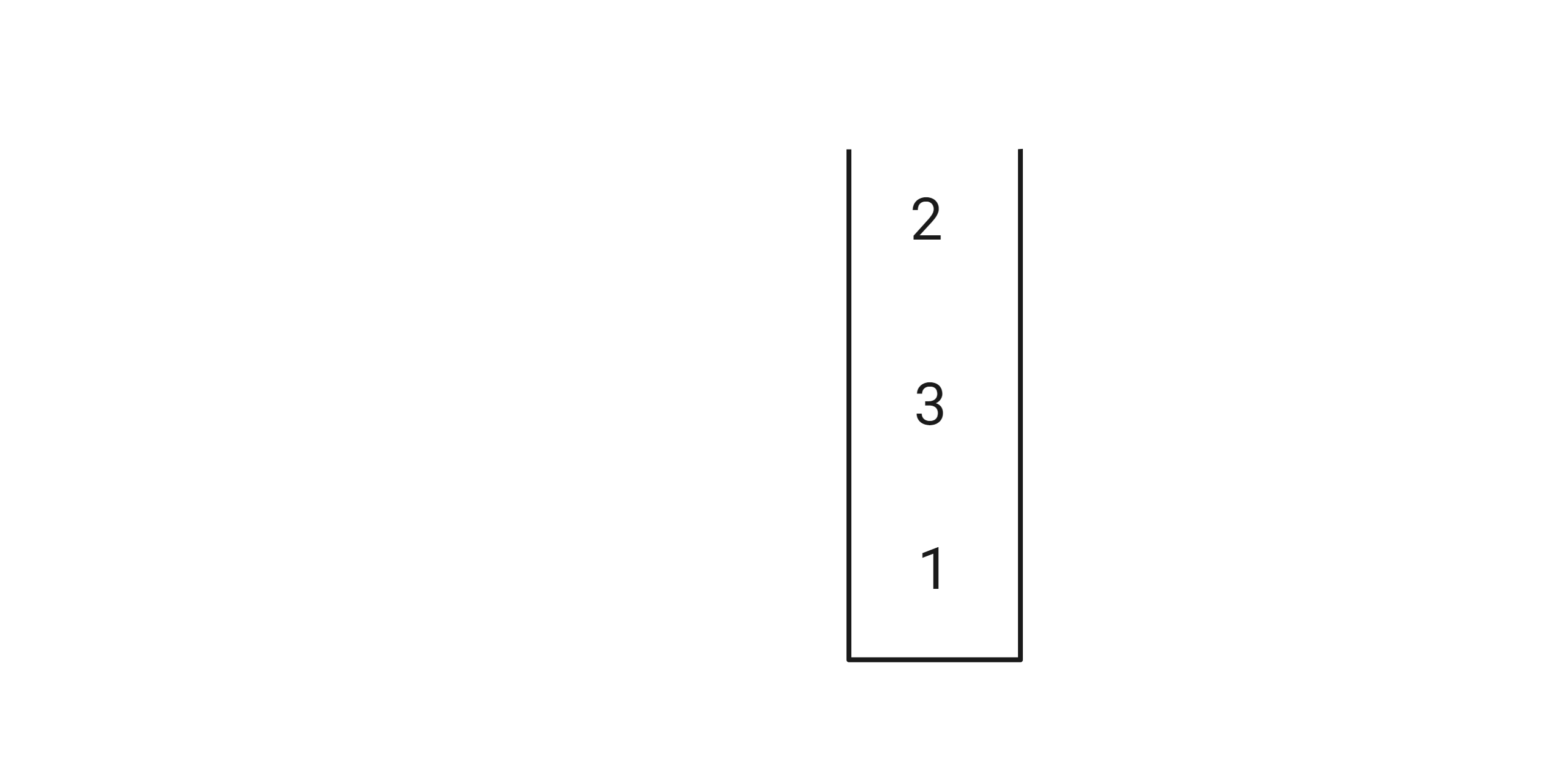# Sort Stack

Posted: 9 Mar, 2021
Difficulty: Easy

## PROBLEM STATEMENT

#### You are given a stack ‘S’. Your task is to sort the sack recursively.

##### Note:
``````Looping through the stack is not allowed.
You need to return a stack that is sorted in descending order.
``````
##### For example:
``````Given stack S = 1 3 2
The output will be 3 2 1 since it is the sorted order.
``````
##### Input format :
``````The first line of input contains an integer ‘T’ denoting the number of test cases.
The second line of each test case contains a single integer ‘N’, denoting the stack size.
The next line contains ‘N’ space-separated integers denoting the elements of the stack, the last element of the input being the top of the stack.

If the input is 1 3 2 then the input stack will look like this :
``````##### Output format :
``````For each test case, print the stack in descending sorted order.

The output of each test case will be printed in a separate line.
``````
##### Note:
``````You do not need to print anything. It has already been taken care of. Just implement the given function.
``````
##### Constraints:
``````1 <= T <= 5
1 <=  N <= 2000
0 <= S[i] <= 1000

Where ‘T’ is the total number of test cases, and 'N’ is the size of the stack, and 'S[i]' is the element of the stack.
``````Approach 1

The main idea is to remove the elements recursively from the stack until the stack becomes empty and then insert those values (from the call-stack  [A call-stack is the stack that is made in the memory when recursive-calls are made. ]), back into the stack in a sorted position.

• In each call, remove the top element from the stack.

• Make a recursive call to the function sortStack.

• After the call, insert the element in the stack in a sorted fashion.

• This can be done by removing the top element from the stack until the topmost element is greater than the current element that we want to insert.